Volume 5, Issue 2, January 2019, Pages 37-49

## Accurate Forecast Improvement Approach for Short Term Load Forecasting Using Hybrid Filter-Wrap Feature Selection

DOI: 10.18775/ijmsba.1849-5664-5419.2014.52.1004
URL: http://dx.doi.org/10.18775/ijmsba.1849-5664-5419.2014.52.1004

### 1 Samuel Atuahene,  2 Yukun Bao, 3 Patricia Semwaah Gyan,  4 Yao Yevenyo Ziggah

1 2 Center for Modern Information Management, School of Management, Huazhong University of Science and Technology, Wuhan-China
3 Faculty of Earth Resources, China University of Geosciences, Wuhan-China
4 Department of Geomatic Engineering, University of Mines and Technology, Tarkwa, Ghana

Abstract: Accurate hybrid ﬁlter– wrap approach is quite important for short term load forecasting as it not only improve forecasting accuracy performance, but also could effectively avoid converging prematurely. The importance of input selection-features is an essential part to develop models. Currently and dynamic surroundings, energy demand, quantity and values are becoming unpredictable and progressively volatile. Increasing amount of decision-making procedures in the industries in terms of energy require a wide-ranging outlook of the uncertain forthcoming. This paper explains the selection method for the proposed hybrid filter-wrapper whose primary composition includes Personal Modular Impactor (PMI) based filter technique and the Firefly Algorithm (FA) based filter wrapper. The filter wrapper planning technique involves the selection of the best corresponding inputs by a predefined model-free technique that measures the specific relationship between the output selection and the input variable. FA wrapper based technique is more useful compared to the filter procedure. Modular Impactor (MI) is a technique mostly preferred by individuals to measure the dependency of variables and commonly used to select input features and in other key fields. hybrid filter

Keywords: Load Forecasting, Energy Forecast, Personal Modular Impactor, Firefly Algorithm

Accurate Forecast Improvement Approach for Short Term Load Forecasting Using Hybrid Filter-Wrap Feature Selection

# 1. Introduction

Lord forecasting in recent years, dynamic surroundings and values are becoming unpredictable and progressively volatile. Increasing amount of decision-making procedures in the industries in terms of energy require a wide-ranging outlook of the indeterminate forthcoming. Numerous decision makers are depending on probabilistic-predictions to enumerate those uncertainties, instead of point forecasting. Thereby, using a term like ‘‘energy-forecast’’ which will be referring ‘‘forecasting in the energy sector’’, including the prediction of the supplies, electricity cost as well as demand. Also water, gas and renewable energy resources. Probabilistic predictions are possible to be in numerous forms, for instance, from quantile to full density forecasts, as well as for multiple definite functional data or variables. The essentials for probabilistic-energy predictions in terms of business spread through operating and planning of the entire energy value chain. Many researchers aiming at energy forecasting have published numerous articles over. A handful of recent literature review papers have presented more inclusive views for numerous subdomains of energy predicting, for example, prediction of wind power, prediction of electric load which was presented by Hong & Fan, and prediction of price of electricity by Weron et. al, 2014. As there are variances amongst the sub-domains, there happen to be couple of mutual challenges: hybrid filter

(1) Probabilistic predicting procedures. Diverse sub-domains in predicting energy include diverse stages of development in terms of probabilistic prediction. Wherein probabilistic predicting techniques, for instance, the ones studied by Gneiting et.al 2014, are relevant to load forecasting? What exact methodologies are actually appropriate for an assumed sub-domain? What is the finest method for generating input situations? What methods are effective for the generation of probabilistic forecasts? What are the processes for stimulating residuals? hybrid filter
(2) Integration. A probabilistic predicting procedure is able to be divided into numerous components, for instance, modeling, generation of scenario, and post-processing. An ideal result of a single component could not be the ideal one for the whole development. The encounter is to assimilate the numerous steps with the aim of obtaining high-quality probabilistic prediction. A comparable integration challenge has also been in recognition in GEFCom,2012 (Hong et.al, 2014).
(3) Cleansing of Data. The practical data for the prediction of load are not at all times clean. Cleansing of data remained acknowledged as a common challenge in the Global Energy Prediction Competition in 2012 (GEFCom2012), that is focused on point prediction (Hong et. al, 2014). Cleansing of data is still an encounter for probabilistic-energy predicting.
(4) Prediction mixture. Mingling a set of prediction points typically outcomes in further precise and robust predicting points. Should a comparable probabilistic load predicting concept be accepted? Must point prediction be syndicate to output a new forecasting point initially, hence generating probabilistic forecasts, or Can there be a combination point prediction in giving probabilistic forecasts? In what way can a probabilistic prediction be combined in generating an improved probabilistic forecast?hybrid filter
In actual sense, energy predicting involves an extensive variety of predicting difficulties in the utility industry, for example, generation forecasting, price predicting, load predicting, demand response predicting, and many more. hybrid filter

# 2. The Technique for the Proposed Hybrid Filter-Wrapper

This particular part explains the selection method for the proposed hybrid filter-wrapper whose primary composition includes Personal Modular Impactor (PMI) based filter technique and the Firefly Algorithm (FA) based filter wrapper. The filter wrapper planning technique involves the selection of the best corresponding inputs by a predefined model-free technique that measures the specific relationship between the output selection and the input variable. FA wrapper based technique is more useful compared to the filter procedure. Modular Impactor (MI) is a technique mostly preferred by the individuals to measure the dependency of variables and is commonly used to select input features and in other key fields. hybrid filter
hybrid filter
There is no assumption in PMI method on the nature of certain relations between the input variables. Thus, MI is often utilized and more accurate in determining the dependency of input variables that possess nonlinear relations compared to estimation (for instance correlation analysis and principal component) that consider the specific linear relations between the respective input variables. This resulted in the main issue of redundancy since MI cannot account for a candidate’s variable interdependency. hybrid filter
hybrid filter
PMI refers to the statistical old version method that is nonlinear in nature and based on partial correlation, even though its primary use has been shown in such fields as water resource analysis, humidity, and rainfall analysis, environmental modeling, and streamflow forecast.
hybrid filter
According to Bowden et al. (2005), the estimation of probability densities involves the application of the city block distance kernel. The x variable and y variable denote the residual data available in variable x and y considering the effect caused by predictor(s) z. The input variable with the most PMI value is included as a new predictor of the performance of characteristic and feature selection centered on PMI. The following is a brief outline of the procedure for the PMI centered input attribute main choose for our proposed technique.
hybrid filter
Step No 1- Let Z represents the number of particular input values that are selected and explained as a null sector at the beginning of the algorithm and Z out represents a set of the input main variables not selected.
Step No 2- Approximate the PMI scorers between the equivalent based on variable Y and every variable in Z out, depending on the pre-determined determiner set Z.
Step No 3- Determine the output variables and input variables that possess the highest PMI score in preceding procedure.
Step No 4- Boot-strap mostly from the total values of z best to result in a selected sample which can be largely concluded to satisfy the set assumption of interdependence between y and z variables of the Personal Modular Impactor scores in the resampled sample. This step should be repeated multiple times to regenerate a PMI scores distribution and puts down the resultant 90th percentile in the Personal Modular Impactor score.
Step No 5- Go back to stage 2.
Step No 6- Stop the process when all the essential inputs have been largely determined.

# 3. The FA Main based Wrapper Technique

The best input subset to be utilized in wrapper techniques is typically selected about the model build the main approach that is universally the resultant error of the forecast model. The study selects the mean absolute percentage error (MAPE) and the scale-free error criteria as the ways of the selection process. As previously discussed, the prediction models computation with wrapper technique is often costlier. Therefore, there is a necessity for an effective searching algorithm designed to determine a unique characteristic subset within the space of the massive attribute subset.
hybrid filter
The algorithm applies MAPE and FA feature selection to perform its search process through a particular number of individuals referred to as fireflies. These specific fireflies are often updated basically from one step to the other. Each and every firefly relates to a particular attribute subset. In the approach, the search space often modeled to represent a multi-dimensional Boolean region while the nature of the feature selection is largely considered. An individual representation serves as an example in Fig 1.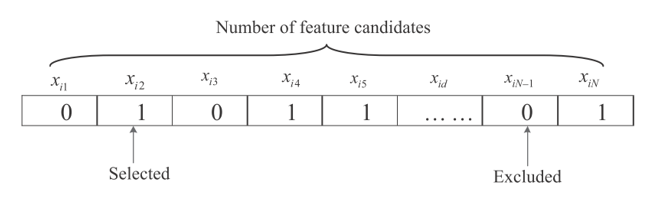Figure. 1 Individual representation as a binary bit string.

Every firefly in the approach is practically assumed to have a particular attraction to other fireflies no matter their set condition, sex, behaviors and the main pleasing is about their visibility and fireflies light intensity. The attractiveness of a given Firefly is referred to as current minus MAPE. Implying that the miniature MAPE Firefly (that is in relation with specific attribute subset) receives the bigger chance of survival.
Firefly movement i to be attracted by another Firefly j that is more attractive can be represented by Eq (1).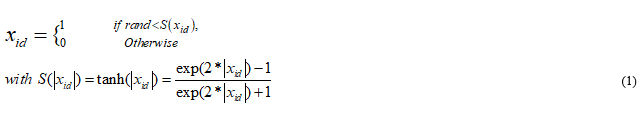Where rand refers arbitrary selected integer generator which is identically selected and equally dole out [0, 1], and represent the main transfer function of the hyperbolic tangent sigmoid.

## 3.1 Our Suggested Hybrid Feature Selection Method

The STFL distinctive selection comprises of two objectively. The first objective is to locate the essential features together with the loads that can be able to appropriately improve the future forecasts of the loads. The second objective is determining a minimal group of key attributes with minimum superfluity that can greatly minimize the costs of computation of the entire model procedure without changing the precision of the forecasting process. A high quality main filter-wrapper technique for STLF is advanced to tackle the goals outlined. Firstly, the PMI-based technique is utilized to remove the number of repetitive features as well as irrelevant features to minimize the complexity witnessed in the search region. Then, according to the minimized attribute subset attained by the Personal Modular Impactor-based filter technique, a Filter Approach-based wrapper approach is utilized to determine a minor group of characteristics with the forecasted hybrid technique thus takes significant impact of the accuracy of filter method and the usefulness of the wrapper method to improve the advantages of every method. Fig 2 is an illustration of the suggested main hybrid filter-wrapper attribute selection method.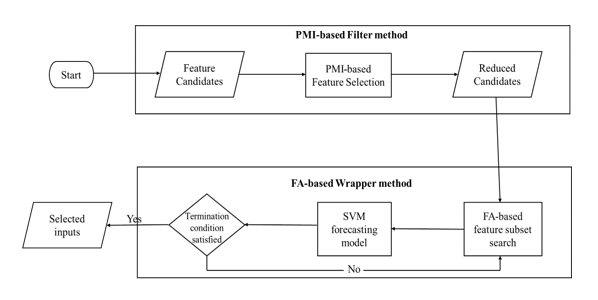Figure. 2: A proposed hybrid feature selection technique flowchart.

# 4. Setting-up the Experiment

## 4.1 Description of Dataset

To validate the future prediction of the success of the proposed main hybrid filter wrapper feature characteristic selection technique for STLF utilizing three actual datasets, SVR obtained from a prominent electrical utility in North-America and GEFcom 2014, were utilized for validation and verification purposes in this particular study. The primary dataset involves consideration and analysis of the North-American electric utility relating to the hourly temperature in farads ((°F) and the hourly rate in (MW). The data collection period ranges from January 1, 1986, to October 14, 1994. Four given months (i.e., April, January, October and July) were selected during the year 1991 as the relative testing periods. The specific months selected was based on the representation of winter, autumn, summer, and the changing durations of the specific year and therefore, produce an excellent approach to be used to test the selected method.
hybrid filter
Data obtained from the preceding three months then similar particulars obtained in the previous years was utilized as the reference group to determine the predicting selected model. Even though many data used to compute the results was obtained from the historical information, the study found out that including more data to more computational costs and this does not result in the improvement of the forecasting accuracy. For the study to replicate the real world applications/synopsis, the datasets were mainly examined to provide a completely distinct and independent relationship to the given training groups of data and were not used in the learning process.
hybrid filter
The selected immediately after the first database amounts to each hour temperatures (in °Farads) obtained from 12 weather stations located in some regions of the United States and hourly loads (in kilowatts) obtained from 21 distinct geographical areas. The initial data was organized for the use of the forecasting load track of GEFcom2013. After the completion of the database, it was published in the appendix found in the Hong et al. (2014) ranging from the period January 1, 2005, to July 7, 2009. The key objective of this main research is to determine the suggested selected filter-wrapper attribute selection techniques performance against many well-analyzed and laid down techniques for future load prognostication and prediction instead of contributing in the process of competition. Thus, the study focused on the next day forecast every hour load for the previous month (July 2, 2009, to July 30, 2009) and ignored the task of a particular competition. The research determined that the demand patterns change significantly over the years. Thus, the three months were selected over the last three years to be used as the reference set to assess the prognosticating predicted approach.
hybrid filter
The researchers have to study for the specific weather stations for every specific area before developing the model since the corresponding locations of weather base, and this particular dataset is not identified. Referring to Ben & Hyndman (2015), an individualistic selected group of data for validation was utilized to determine the type of station to be used for every region. The main weather camp that possesses the best result was isolated to determine the prognosticating and prediction approach for the month used for the testing process. Some processing procedures and methods were required before modeling the datasets. For instance, various unaccounted load amounts in the original data group were occupied by the average values of the neighboring selected values. There is a significant increase in demand in the year 2009 for zone 11 available in the secondary selected dataset. Utilizing the information from Ben & Hyndman (2015), we calculated the criterion utilized previously and then after the increase, and then the distinction was recorded and eliminate the jump in the year 2009.
hybrid filter
Following Charlton & Singleton (2014), some outliers in the process were detected and removed. To avoid scaling issues and unit issues, every input variable in the study was linearly identified to the ultimate range starting from [0, 1] utilizing their particular selection of minimum values and maximum values correspondingly. The forecasting predicted value amounts will be rescaled backward due to the reserves of forecasting performance, and the linear transformation is computed based on the preliminary scaling of the collected-data. In order to develop the required pool of features representing candidates to the prediction approach, by obtaining the electricity utilization load from the beginning set dataset to act as a reference, the study determined some of the features of the loads selected after every one hour.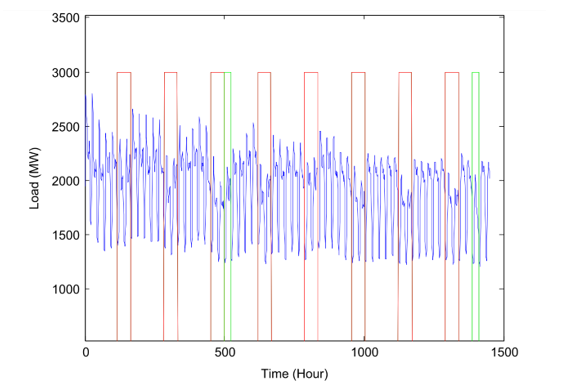Figure. 3: Shows the Hourly loads from May 2, 1991, to August 6, 1991

Fig. 3 shows the predicted load after every one hour from May 2, 1991, till August 6, 1991. The figure portrays the load at most of the weekends and the set holidays (Christmas Day, Labor Day and Freedom Day) are represented with green rectangular shapes and red rectangles consecutively. Therefore, with reference to the figure shown below, it’s evident that the current load utilization and demand possess a number of seasonal patterns comprising of weekly and daily periodicity. Moreover, the load levels at weekends and the set holidays are much lower concerning the working days. This result concludes in the sense that, the demand of load is often influenced and greatly affected by the calendar days. Nevertheless, it is a very challenging task to forecast on holidays since the typical conditions are rare and distinct from regular workdays and other holidays. In this study, the researchers took the weekends and holidays to be the same for simplicity purposes.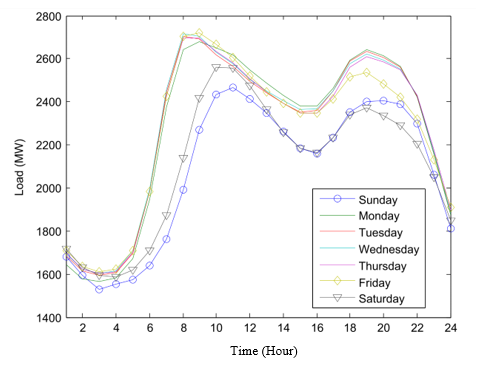Figure. 4: Loads witnessed after every one hour during each day of a week.

Fig. 4 portrays the mean hourly-predicted load for the same day for every day for a period of one week. There is a variation in the load from one hour to another due to the consumers’ expected behavior. Also, it is very simple to determine that the expected curves available on all working days, without considering Friday, since it possesses the same magnitudes and shapes. This act as proper proof that the working days have higher load demands than the weekends. However, Friday has a load level between levels of weekends and working days. Moreover, the relationship that exists between the temperature and the load demand is portrayed in Fig. 5 below, which led to the observation of a nonlinear relationship between them. This is a confirmation that the temperatures are worth applying to the input variables.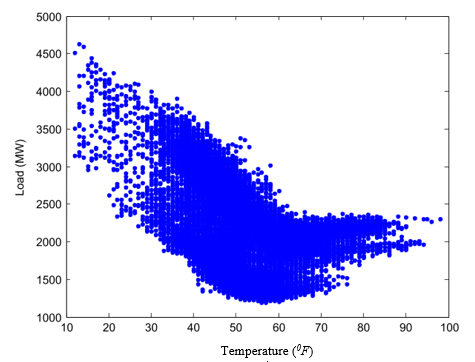Figure. 5: Relationship between temperature and load.

Putting into consideration the short run behavior and each day periodicity features of each one hour loads, this study selects each hour load’s amounts of the preceding 12 hours and same hours in the last week as the prime inputs integers of a certain prediction model. There was an addition of temperature variables every period region for which the test load was added with the predicted temperature for a particular forecasted hour. The average measured temperature value on a given hour and date within the previous five years was utilized to represent the predicted temperature. Many, who participated in GEFCom2014, for instance, Ben & Hyndman (2015) and Charlton, used the average temperature to represent the predict in their approaches. Besides of the scenario, each hour and daily events indicators were utilized and used to codify the hours of a day as well as seven days per week. The candidate set of inputs variables to be utilized to predict the value of the load L (t) can be summarized in the diagram below in Eq. (2). hybrid filter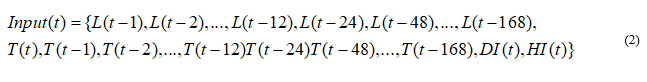Where L (T – i) means the lag behind load of ( t – i); where T (t – i) indicates the previous temperature of t – i; T (t) b representing the condition having the previous period of 0, which represent the predicted.
hybrid filter
The temperature for a certain predicting hour; and HI (t), DI (t), signify each hour and daily event results consecutively. DI (t) results to -2 for most of the weekends and set public, days set 0 for Thursday, and 1 for all days for working with the exception for Thursday. HI (t) amounts to 1, 3, 4, 5, 6… 25 for the equivalent hours. In total, there are (11+6) + (12+6) + 2=41 main inputs in the set. Based on the same predictions, the input variables pool for the load numbers in the following dataset group (except region 8) is referred and shown by Eq. (2) at the time of modeling the load series representing load 9. Region 9 of the second set of data contains extremely different patterns of demand that do not seem to be corresponding to the values of the temperature. Thus, the researchers excluded the information on the temperature Eq. (2) at the period of changing the load series located in Region 9.

## 4.2 Completion Metrics

This particular study proves the forecasting model performance by utilizing the MAPE in Eq. (3) and MASE in Eq. (4) respectively. These can be defined as: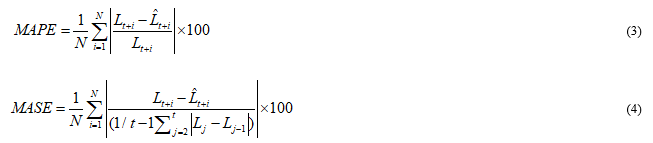Widely used standard of measurement, which measures the difference in percentage between the predictable and estimated standards. In which N denotes the prediction horizon, is the is the actual value at period t + i , and 20 predicting value at period t+ i. As proposed in this article, the next day (24 hours) the short-term predicted load was predicted repetitively, therefore, numerous prediction periods N is equal to 24. The MAPE one of the most extensively used standard of measurement that measures the given percentage difference between the expected and estimated values. The small values obtained in MAPE integers represent the closest predictions to the real integers. Thus, the MASE refers to a reduced error which is particularly scaled by a unique predictive model. The value is often less than one particularly if the prediction is much better compared to the other technique, and the lesser the MASE, the best the results of the prediction relating to the unique technique. The MASE is often the most highly advised measure since it is less responsive to be used by the outliers and simple to interpret.

# 5. Identified Counterparts and their Implementations

There are five other feature selection techniques selected to prove the benefits that result from the proposed wrapper technique for STL utilizing SVR and used as reference points for purposes of comparison. These counterparts and the approach proposed are written and abbreviated as shown below:
(1) Full-The SVR prediction and predicting model with all the required inputs.
(2) MI-SVR approach with MI-based attribute selection method (Daraeepour, 2008).
(3) Two Stage- SVR approach with two-stage attribute selection.
(4) PMI-SVR method applied composed of the PMI based technique for actual characteristic-identification.
(5) FA-SVR approach with implemented FA-based attribute selection.
(6) H-FW-SVR approach with the suggested hybrid filter-wrapper procedure for attribute selection.
hybrid filter
In the abbreviations above, ‘Full’ functions even when there is no feature selection in place. Previous studies provide ‘MI’ and ‘Two Stage’ as the existing techniques studies (Amjady & Daraeepour). ‘MI’ is designed to identify irrelevant features only that are based on MI. ‘Two Stage’ is designed first to remove the features that are irrelevant in the MI and then remove the respective characteristics with respect to the value of MI between the two main integers. PMI and FA are two techniques that are mentioned in the previous sections. ‘MI’, PMI and ‘Two Stage’ represent the filter techniques. H-FW refers to the suggested hybrid wrapper approach. FA represents a wrapper method.
hybrid filter
SVR has been continuously applied as a forecasting modeler in all the methods mentioned above. One essential feature in SVR training and implementation involves the setting of a certain hyperparameters and kernel functions. This experiment identified the selected reference function to represent the kennel function after the main experimentation. The efficiency of SVR is not a big concern since the data sequence is not lengthy. Therefore, we conduct the matrix search to the parameters C, and Utilization of SVR was evaluated on the period of one-day ahead prediction. The multiple stages of head forecasts were determined via recursion.
hybrid filter
There is a note to be taken on the unit variable to be used to predict the other hour load although, there are several studies that investigated the strategies used for many steps ahead predicting the research is of no concern to us since we utilized recursion strategy only in the study. All the experiments conducted during the study were carried out in MATLAB 2014 utilizing the computer with the features Compaq Core 4 Duo Central Processing Unit T6850, 6.00 Gigahertz, and 4 GB Random Access Memory. The difference of the 6 SVR methods was the difference in the methods used. The values containing ‘MI’ and ‘Two Stage’ were specifically s group based on their initial preferences.
hybrid filter
The program loading number indicated in PMI was specifically located to twenty. The population size of filter parameters was initially at 31, attractiveness 001, coefficient 121, and stopping procedure set as indicated; some changes amount to 151, or there is the lack of advancement in the fitness for almost twenty respective selected steps. Finally, we did not include each of the models 12 times, and the results of improvement mean reported.

## 5.1. Results – The North American Electrical Utility

Table I summarizes the value of the results of feature selection by utilizing six main techniques for predicting hourly loads reported in January 1991, as a result of the space constraints. In the table, some selected inputs (‘No.’), List of inputs selected (‘Input (lags)’, time spent for feature and the characteristic identification (‘Tfs (minimum)’, the time spent for forecasting utilizing inputs selected (‘Ttf (minimum)’), time spent for the whole procedure (‘Tall (minimum)’), and MAPE of the relating (‘MAPE’) as portrayed. The value with that has the smallest number is written boldly. As indicated in the table, although all the values of imputing dataset were used for developing the predicting model, the results of the forecasting step of full is worst, with higher MAPE compared to others, which necessitates the feature selection of STLF. This is as a result of the irrelevant features that cause the model to overfitting. The other 5 main feature selection techniques involve reduction in dimensionality, and the space models are then obtained without necessarily reducing the prediction accuracy.
hybrid filter
‘MI’ and ‘Two Stage’ generates relatively high amount of inputs among the three filter techniques than ‘PMI’. This occurs since MI-based approach removes the unwanted characteristics by calculating the MI value that is between each specific variable and output. This means that there are several repetitive features used in the identified inputs by utilizing ‘MI’. ‘Two Stages’ removes features that are repetitive in the next step but it does not consider the already predictive values. On the other hand, ‘PMI’ obtains essential characteristics (those that are so important and not redundant) stage by stage based on the partial information given. ‘FA’ has the relatively higher amount of inputs compared to the hybrid ‘H-FW’, and PMI’ which is primarily difficulties caused by the procedure in turning the 41-proportional search region.
hybrid filter
The mathematical calculation time (‘Tfs(min)’ shown in Table 1 takes more time due to the presence of the SVR and the search region of the filter which is high dimensional in nature. ‘H-FW’ can identify the ideal characteristic subset well organized due to the small search region. The more inputs values selected increases the period for forecasting and training (‘Ttf(minimum)’) H-FW based model is often higher in terms of other counterparts, but this is allowable for daily conclusion making. Fig 6 illustrates the input subset selection using other feature selection methods comparing the accuracy of the prediction per month.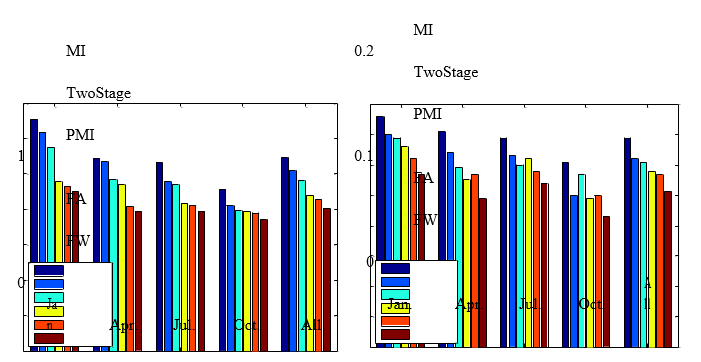Figure. 6. MAPE and MASE compared for dissimilar models based on the first Case.

In Table 1, the values of the reduced input sets enabled an interpretation in an intuitive manner. For instance, the previous 12 hours involves several recent hour loads (preceding 1-6 hours) that relate to the current loads, are essential for prediction. Instead of utilizing the past various hourly loads, the loads representing the same hour are essential in forecasting, including the lagged loads obtained from the previous 36 and 168 hours. Also, the condition at the one hour if forecasting (T:1) has been predicted as one of the inputs for the 5 attribute selection criterion that portray that Fig.7 presents the average predicted accuracy for every week during the testing period. The results confirm the supremacy stated in the proposed main hybrid-filter wrapper technique based on forecasting method and other techniques in a week. Moreover, it is noted that load forecasts represent higher errors in weekends than in workdays. This is due to particular load patterns of Friday and weekends are the district to normal days, which Fig.4 has also indicated.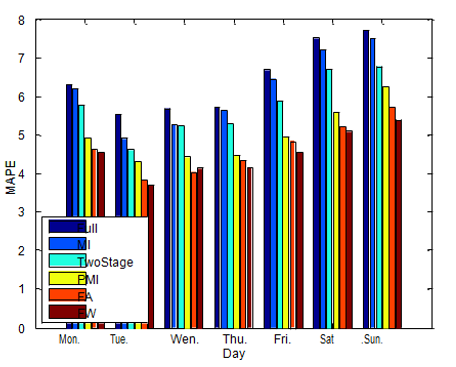Figure. 7: Average prediction of the MAPE for each day of the week during the testing period

Fig. 8 shows the illustration of the forecasting respective performance of the model proposed an example of the given load and the predicted hourly load. It represents the actual load, the prediction, and errors in March 1992. The outcome depicts the predicted values being quite close to the main values, and minimal mistakes are visible.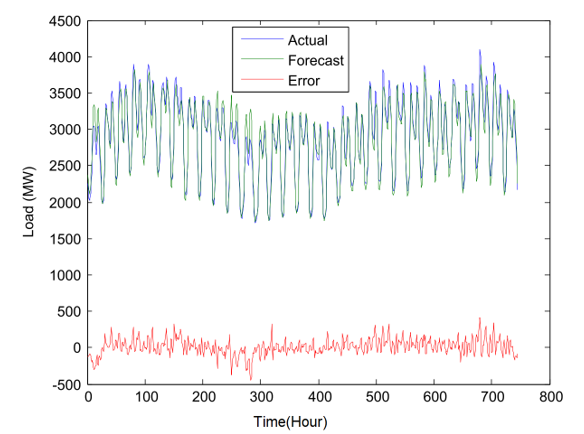Figure. 8: Electricity Load versus time in hours

Table 1: Results of six different techniques analysis for prediction of loads in January 1991 per hour.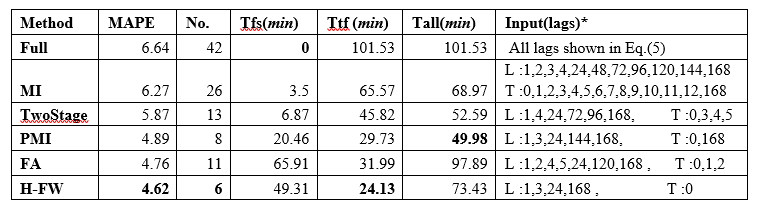NB: L indicates Load, T indicates Temperature. DI and HI are all selected.

Table 2: MAPE (%) Comparison in terms of different models based on Case 2.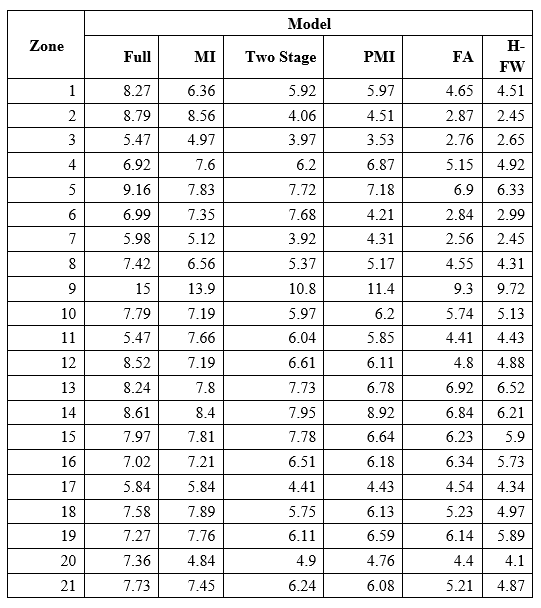## 5.2 Statistical Test Analysis

Two-stage statistical analyses are utilized to prove if the corresponding outcome acquired by the 6 predicting models on the 4 months that were put to the test shows that Case 2 was significantly different based on the Case 1 and 20 zonal loads. The statistical analysis utilizes the freedman tests and stepwise, both of which involve strong statistical evidence, used for juxtaposition by a combination of the data obtained from the two main occurrences. The Friedman inspection is used to calculate the mean rank got by each model beyond both the data, to determine if remarkable differences are available between the predicting approaches based on the values of the mean ranks. Table 2 shows the differentiation of the MASE for various models in relation to Case 2. Table 3 shows the Freadman rank and the p-value of Davenport and Iman test MAPE. The part highlighted in bold shows the best technique with the lowest rank. The table portrays a p-value of Davenport and Iman test to be lower than the significance level 0.05. This shows the statistical difference in the results of the six models.

Table 3: Differentiation of the MASE for various models in relation to Case 2.To determine the statistical importance between the results obtained and the other main perspective, a step wise test, called the Holon test, was utilized at the important stages of 0.2 and 0.06. Table 4 shows the results of the first 4 hypotheses that are prohibited (at the importance levels of 0.05) because their p-value is less than the value of the adjusted amounts (alpha/i). the last approach FA is so small that the result is rejected at 0.01 level.

Table 4: Friedman’s experiment outcome for diverse predicting models.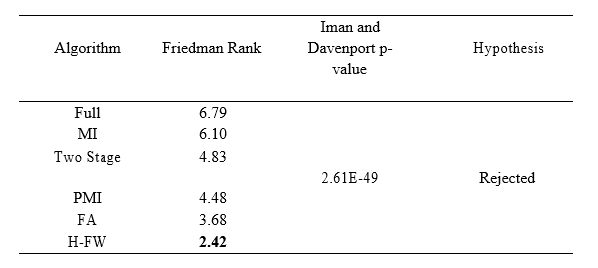Table 5: Friedman’s experiment outcome for diverse predicting models.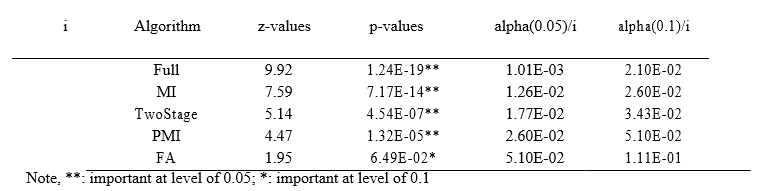# 6. Conclusion

Attribute selection is an important step in STLF to simplify the interpretation of dataset and process of the learning forecasting model. This study proposes the main high-quality filter-wrapper attribute selection technique to address the issue relating to the characteristics selection issue. The suggested hybrid technique comprises of a FA-based wrapper approach and a PMI filter technique. Firstly, the filter procedure is utilized to do away with the redundant and irrelevant features, to produce input values. A wrapper technique is applied to the minimized subset to determine the small set of features with large prediction accuracy. Exploratory results have indicated that the approach suggested can be able to identify leases input integers compared to other old methods and it is a more efficient wrapper method.
hybrid filter
Thus, the hybrid filter-wrapper technique is an excellent alternative for the process of attribute selection in STLF. Therefore, in this particular study, the most common input values only were greatly considered in the model choosing the procedure. Forthcoming work needs to be done to analyze other essential factors and more lagged factors to improve prediction accuracy. Since the load methods of remarkable days are distinct from regular weekdays, leading to a particular prediction model for the given special days is worth research attempt. Other areas to be done in future research comprise an extra comprehensive juxtaposition of the approach suggested with other established highest-level models, and in the process apply the proposed technique for future long-term electricity price forecasting and load prediction.

# References

• Charlton, N., Singleton, C., 2014. A refined parametric model for short term load forecasting. Int. J. Forecast. 30, 364-368
• Amjady, N., Daraeepour, A., 2009. Mixed price and load forecasting of electricity markets by a
new iterative prediction method. Electric Power Systems Research 79, 1329-1336.
• Guyon, I., Elisseeff, A.e., 2003. An Introduction to Variable and Feature Selection. Journal of
Machine Learning Research 3, 1157-1182.
• Amjady, N., Keynia, F., 2011. A New Neural Network Approach to Short Term Load Forecasting of Electrical Power Systems. Energies 4, 488-503.
• Bao, Y., Xiong, T., Hu, Z., 2014a. Multi-Step-Ahead Time Series Prediction using Multiple-
Output Support Vector Regression. Neurocomputing 129, 482–493.
• Bao, Y., Xiong, T., Hu, Z., 2014b. PSO-MISMO Modeling Strategy for Multi-Step-Ahead Time
Series Prediction. IEEE Transactions on Cybernetics 44, 655-668.
• Amarawickrama, H.A., Hunt, L.C., 2008. Electricity demand for Sri Lanka: a time series analysis. Energy 33, 724-739.
• Guyon, I., Elisseeff, A.e., 2003. An Introduction to Variable and Feature Selection. Journal of
Machine Learning Research 3, 1157-1182
• Ben Taieb, S., Hyndman, R.J., 2014. A gradient boosting approach to the Kaggle load forecasting competition. Int. J. Forecast. 30, 382-394.
• Bermejo, P., Gamez, J.A., Puerta, J.M., 2011. A GRASP algorithm for fast hybrid (filter-wrapper) feature subset selection in high-dimensional datasets. Pattern Recognit. Lett. 32, 701-711.
• Bowden, G.J., Dandy, G.C., Maier, H.R., 2005. Input determination for neural network models in water resources applications. Part 1 – background and methodology. J. Hydro. 301, 75-92.
• Chandrasekaran, K., Simon, S.P., 2013. Optimal Deviation Based Firefly Algorithm Tuned Fuzzy Design for Multi-Objective UCP. IEEE Trans. Power Syst. 28, 460-471.
• Hahn, H., Meyer-Nieberg, S., Pickl, S., 2009. Electric load forecasting methods: Tools for
decision making. Eur. J. Oper. Res. 199, 902-907.
• Charlton, N., Singleton, C., 2014. A refined parametric model for short term load forecasting. Int. J. Forecast. 30, 364-368.
• Chen, B.J., Chang, M.W., Lin, C.J., 2004. Load forecasting using support vector machines: A
Study on EUNITE competition 2001. IEEE Trans. Power Syst. 19, 1821-1830.
• Hen, Y., Luh, P.B., Guan, C., Zhao, Y., Michel, L.D., Coolbeth, M.A., Friedland, P.B., Rourke,
S.J., 2010. Short-Term Load Forecasting: Similar Day-Based Wavelet Neural Networks. IEEE
Trans. Power Syst. 25, 322-330.
• Demšar, J., 2006. Statistical Comparisons of Classifiers over Multiple Data Sets. Journal of
Machine Learning Research 7, 1-30.
• Amjady, N., Daraeepour, A., 2011. Midterm Demand Prediction of Electrical Power Systems
Using a New Hybrid Forecast Technique. IEEE Trans. Power Syst. 26, 755-767.
• Douglas, A.P., Breipohl, A.M., Lee, F.N., Adapa, R., 1998. The impacts of temperature forecast uncertainty on Bayesian load forecasting. IEEE Trans. Power Syst. 13, 1507-1513.
• Drezga, I., Rahman, S., 1998. Input variable selection for ANN-based short-term load forecasting.
• Fan, S., Chen, L.N., 2006. Short-term load forecasting based on an adaptive hybrid method. IEEE Trans. Power Syst. 21, 392-401. c Additive Model. IEEE Trans. Power Syst. 27, 134-141.
• A., May, C., Fusai, G., 2010. Functional clustering and linear regression for peak load.
• Hong, T., Pinson, P., Fan, S., 2014. Global Energy Forecasting Competition 2012. Int. J. Forecast. 30, 357-363.
• Hooshmand, R.A., Amooshahi, H., Parastegari, M., 2013. A hybrid intelligent algorithm based
short-term load forecasting approach. Int. J. Electr. Power Energy Syst. 45, 313-324.
• Guyon, I., Elisseeff, A.e., 2003. An Introduction to Variable and Feature Selection. Journal of Machine Learning Research 3, 1157-1182.
Share.